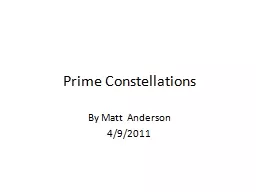# Prime Constellations2016-03-07 46K 46 0 0

## Prime Constellations - Description

By Matt Anderson. 4/9/2011. Prime numbers are integers that are divisible by only 1 and themselves.. P. ={primes} = {2,3,5,7,11,…}. There are an infinite number of prime numbers.. Let . π. (x) be the prime counting function. . ID: 246214 Download Presentation

Embed code:

## Prime Constellations

Download Presentation - The PPT/PDF document "Prime Constellations" is the property of its rightful owner. Permission is granted to download and print the materials on this web site for personal, non-commercial use only, and to display it on your personal computer provided you do not modify the materials and that you retain all copyright notices contained in the materials. By downloading content from our website, you accept the terms of this agreement.

### Presentations text content in Prime Constellations

Slide1

Prime Constellations

By Matt Anderson

4/9/2011

Slide2

Prime numbers are integers that are divisible by only 1 and themselves.P={primes} = {2,3,5,7,11,…}There are an infinite number of prime numbers.

Slide3

Let π(x) be the prime counting function. π(x) counts the number of primes less than or equal to x.

X

π

(x)

1

0

2

1

3

2

4

2

5

3

6

3

7

4

8

4

9

4

Slide4

The prime number theorem states that π(x) grows like x/Ln(x).Specifically,

Slide5

A prime k-

tuple

is an ordered set of values representing a repeatable pattern of prime numbers.

Examples Instances

(0,2) twin primes {3,5},{5,7},{11,13}

(0,4) cousin primes {3,7},{7,11},{13,17}

(0,6) sexy primes {5,11},{7,13},{11,17}

(0,2,6) 3-tuple {3,5,11},{5,7,13}

(0,4,6) 3-tuple {7,11,13},{13,17,19}

(0,2,6,8) 4-tuple {5,7,11,13},{11,13,17,19}

Slide6

A k-

tuple

is said to be admissible if it does not include the complete modulo set of residue classes (

ie

the values 0 through p-1)

of any prime

p≤k

.

The k-

tuples

listed thus far are all admissible, but (0,2,4) is not admissible. Since

0 mod 3 = 0

2 mod 3 = 2

4 mod 3 = 1

The complete set of residue classes mod 3 is {0,1,2}.

The only primes that satisfy this 3-tuple are {3,5,7}.

If the smallest prime is greater than 3 then it will not be possible for all three members to be prime.

Slide7

tuple

that is maximally dense is called a constellation with k primes.

For

n≥k

, this will always produce consecutive primes. Where, n is the smallest prime in the constellation.

Example: The constellation with 2 primes is (0,2).

Example 2: There are 2 constellations with 3 primes.

They are (0,2,6) and (0,4,6).

Slide8

It is conjectured that there are an infinite number of twin primes. Also, it is conjectured that there are an infinite number of primes for every admissible k-

tuple

.

Numerical evidence supports this conjecture.

Slide9

Let π m1, m2,…,mk (x) be the number of (k+1) tuples less than xFor example, consider the 4-tuple (0,2,6,8)The smallest prime has the form 30n+11.

x

π

2,6,8(x)

smallest examples

0

0

{5,7,11,13}

5

1

{11,13,17,19}

11

2

{191,193,197,199}

191

3

{821,823,827,829}

821

4

Slide10

For example

π

2(x) counts the number of twin primes less than or equal to x. Similarly

π

4(x) counts the number of cousin primes less than or equal to x. Twin primes have the from 6x+5 and 6x+7. We know that from divisibility by 2 and 3.

Primes p > 3 must have the form:

p=1 mod 6

or

p=5 mod 6

otherwise, they would be divisible by 2 or 3.

Slide11

To find k-tuples, one must determine the values of a and b inp=ax+b for the smallest prime p in a constellation. One way to do this is by examining Ur# where r# (read r primorial) is the product of the first r primes and Ur# is the set of units mod r#.

rr#12263304210

For example:

U2 = {1}. All prime numbers greater than 2 are odd.

U6 = {1,5}. All primes > 3 have the form 6k±1

U30 = {1,7,11,13,17,19,23,29}

Slide12

The first Hardy Littlewood Conjecture states that every admissible (k+1)-tuple has infinitely many prime examples and the asymptotic distribution is given by:πm1,m2,…,mk(x) ~ C(m1,m2,…, mk)    and w(q;m1,m2,…mk) is the number of distinct residues of m1, m2,…, mk (mod q)

Slide13

The second Hardy

Littlewood

conjecture states that:

π(

x+y

) ≤ π(x) + π(y)

for all x, y with 2 ≤ x ≤ y.

It is believed that there is a counterexample for x=447 and 10

147

< y < 10

1199

Slide14

Examples have been found for constellations of 2 to 23 primes. There are no known examples of constellations with 24 primes.

Slide15

References:

http://www.sam.math.ethz.ch/~waldvoge/Projects/clprimes05.pdf

http://www.opertech.com/primes/k-tuples.html

http://mathworld.wolfram.com/Hardy-LittlewoodConjectures.html

Elementary Number Theory 2

nd

Edition by Underwood Dudley

Prime Numbers: A Computational Perspective 2

nd

Edition

by Richard Crandall and Carl

Pomerance

Slide16

Slide17

Slide18

Slide19

Slide20

Slide21